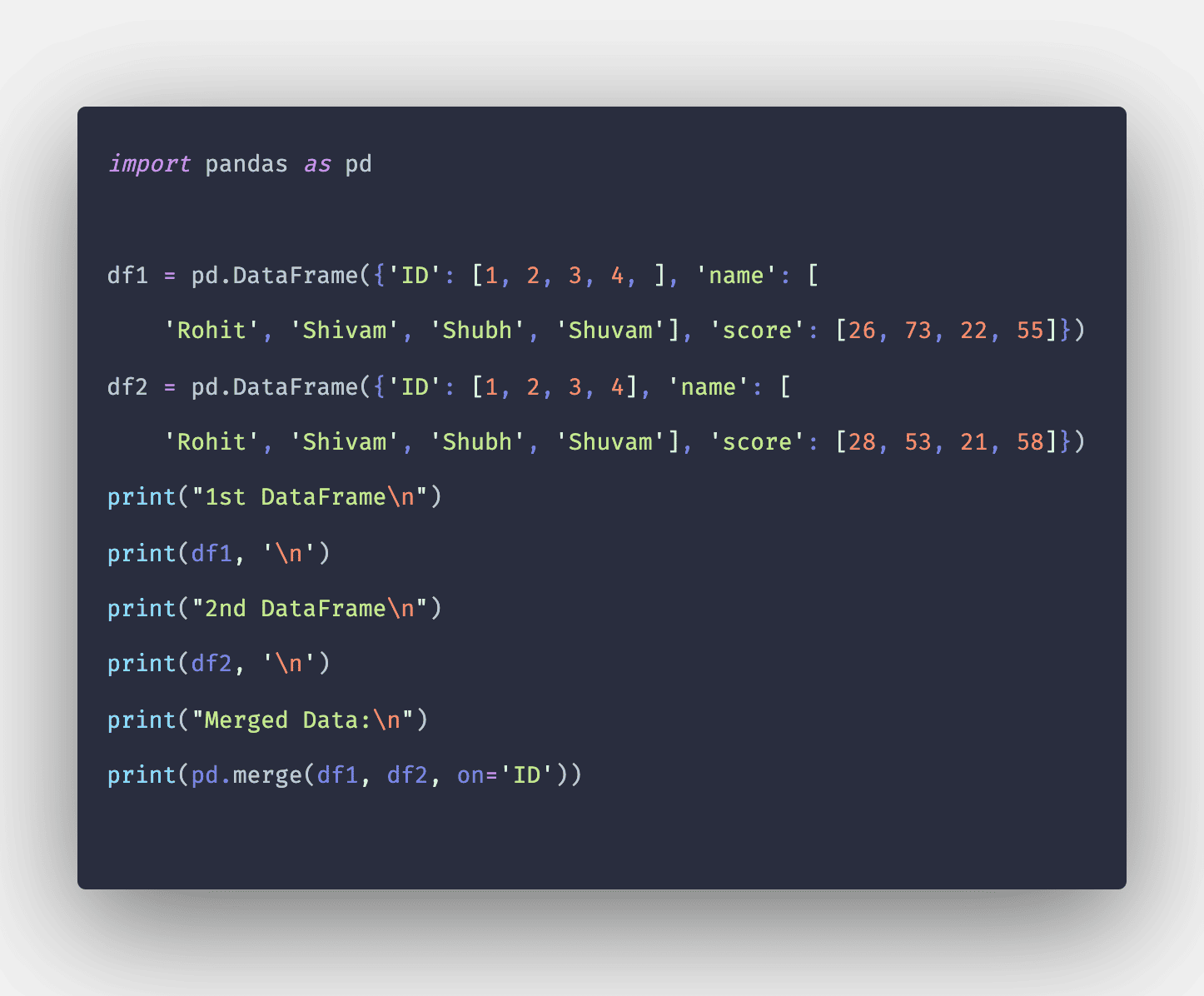# Pandas merge: The Complete Guide

0
46Pandas library provides a single function called merge(), an entry point for all standard database join operations between DataFrame objects.

Pandas library has full-featured, high-performance in-memory join operations idiomatically very similar to relational databases like SQL.

When you have to combine the data objects based on one or more keys in a similar way to a relational database, df.merge() is the tool you need.

## Pandas merge()

Pandas DataFrame merge() is a library method that acts as an entry point for all the database join operations between different objects of DataFrame. The join operation is done on columns or indexes specified in the parameters.

Merging and joining DataFrames is a core process that any aspiring data analyst must master.

Both act differently; if we join columns on columns, then indexes will be ignored. Otherwise, if the joining indexes on indexes or indexes on the column or columns, the index will be passed on.

More specifically, df.merge() is the most useful when you want to combine rows that share data.

### Syntax

```pandas.merge(left, right, how=’inner’, on=None, left_on= None,
right_on = None, left_index = False,
right_index=False, sort=True)
```

### Parameters

Pandas merge() function has nine parameters which are listed below:

1. left = It is the DataFrame object.
2. right = It is also the object of the DataFrame.
3. on = It is the columns to join. It is found in both the left and right DataFrame objects.
4. left_on = It uses the columns from the left DataFrame as keys. It can be either column names or arrays with lengths equal to the length of DataFrame.
5. right_on = It uses the columns from the right DataFrame as keys. It can be either column names or arrays with lengths equal to the length of DataFrame.
6. left_index = It takes Boolean values. If true, it uses the index from the left DataFrame as its join key. In the case of the DataFrame with a MultiIndex, several levels must match the number of join keys from the right DataFrame.
7. right_index = It has the same usage as left_index for the right DataFrame. Just the operating sides are changed from left to right.
8. how = It can have four values left, right, outer, and inner. By default, the value of inner. In addition, it defines the type of merge to be performed.
9. sort = It is used to sort the resultant DataFrame by the join keys in lexicographical order.

### Return Value

The merge() function returns a DataFrame with two merged objects.

### Example program on Pandas DataFrame merge()

Write a program to show the working of pandas merge().

```import pandas as pd

df1 = pd.DataFrame(
{'Lname': ['Rohit', 'Shivam', 'Rohit', 'Shivam'], 'Lscore': [26, 73, 22, 55]})
df2 = pd.DataFrame(
{'Rname': ['Rohit', 'Shivam', 'Rohit', 'Shivam'], 'Rscore': [28, 53, 21, 58]})
print("1st DataFrame\n")
print(df1, '\n')
print("2nd DataFrame\n")
print(df2, '\n')
print("Merged DataFrame:\n")
print(df1.merge(df2, left_on='Lname', right_on='Rname'))```

#### Output

```1st DataFrame

Lname  Lscore
0   Rohit      26
1  Shivam      73
2   Rohit      22
3  Shivam      55

2nd DataFrame

Rname  Rscore
0   Rohit      28
1  Shivam      53
2   Rohit      21
3  Shivam      58

Merged DataFrame:

Lname  Lscore   Rname  Rscore
0   Rohit      26   Rohit      28
1   Rohit      26   Rohit      21
2   Rohit      22   Rohit      28
3   Rohit      22   Rohit      21
4  Shivam      73  Shivam      53
5  Shivam      73  Shivam      58
6  Shivam      55  Shivam      53
7  Shivam      55  Shivam      58```

In the above code, you can see that we have created two DataFrames with different data and then merged the data of both the DataFrames using the merge() function based on their left and right keys.

#### Example 2: Write a program to merge two DataFrames based on a Key (standard in both the DataFrames)

See the following code.

```import pandas as pd

df1 = pd.DataFrame({'ID': [1, 2, 3, 4, ], 'name': [
'Rohit', 'Shivam', 'Shubh', 'Shuvam'], 'score': [26, 73, 22, 55]})
df2 = pd.DataFrame({'ID': [1, 2, 3, 4], 'name': [
'Rohit', 'Shivam', 'Shubh', 'Shuvam'], 'score': [28, 53, 21, 58]})
print("1st DataFrame\n")
print(df1, '\n')
print("2nd DataFrame\n")
print(df2, '\n')
print("Merged Data:\n")
print(pd.merge(df1, df2, on='ID'))```

#### Output

```1st DataFrame

ID    name  score
0   1   Rohit     26
1   2  Shivam     73
2   3   Shubh     22
3   4  Shuvam     55

2nd DataFrame

ID    name  score
0   1   Rohit     28
1   2  Shivam     53
2   3   Shubh     21
3   4  Shuvam     58

Merged Data:

ID  name_x  score_x  name_y  score_y
0   1   Rohit       26   Rohit       28
1   2  Shivam       73  Shivam       53
2   3   Shubh       22   Shubh       21
3   4  Shuvam       55  Shuvam       58```

Here in the above code, we can see that we have merged the data of two DataFrames based on the ID, which is the same in both the DataFrames.

That is it for the Pandas DataFrame merge() Function.